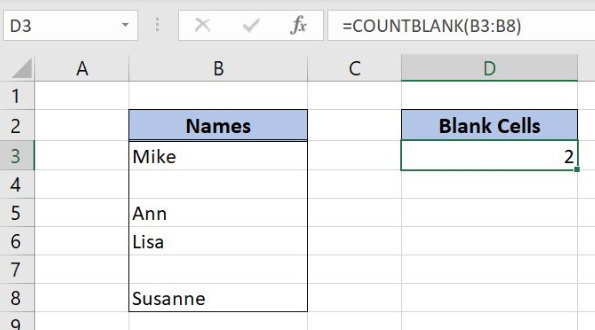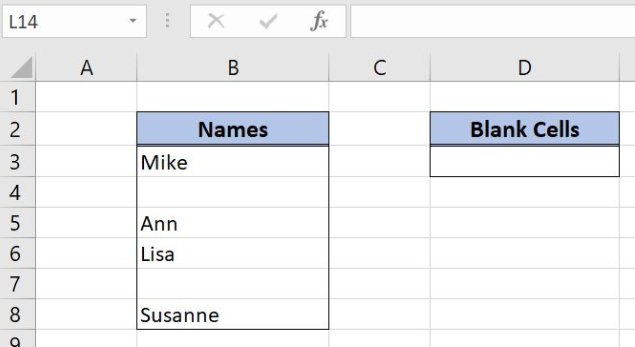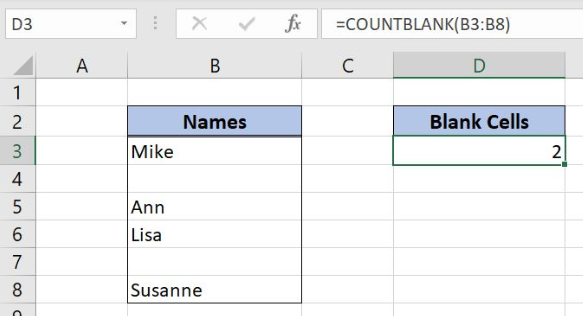Get instant live expert help with Excel or Google Sheets“My Excelchat expert helped me in less than 20 minutes, saving me what would have been 5 hours of work!”

#### Post your problem and you’ll get expert help in seconds.

Your message must be at least 40 characters
Our professional experts are available now. Your privacy is guaranteed.

# Count Cells that are Blank

Excel allows us to count cells that are blank in a range using the COUNTBLANK function. This step by step tutorial will assist all levels of Excel users in counting blank cells in a range.Figure 1. The final result of the COUNTBLANK function

## Syntax of the COUNTBLANK Formula

`=COUNTBLANK(range)`

The parameter of the COUNTBLANK function is:

• range – a range of cells where we want to count blank cells.

Note that the function doesn’t take in a count blank cells as a result of other functions.

## Setting up Our Data for the Examples

In column B (range B3:B8), we have the list of names with blanks. In the cell D3, we want to get the number of blank cells in the range B3:B8.Figure 2. Data that we will use in the COUNTBLANK example

## Count Blank Cells in a Range Using the COUNTBLANK function

In the cell D3, we want to get a number of blank cells in the range B3:B8.

The formula looks like:

`=COUNTBLANK(B3:B8)`

The range parameter is B3:B8.

To apply the COUNTBLANK function, we need to follow these steps:

• Select cell D3 and click on it
• Insert the formula: `=COUNTBLANK(B3:B8)`
• Press enter.Figure 3. Using the COUNTBLANK function to count blank cells in a range

As we can see in Figure 3, there are 2 blank values in the range (B4 and B7). Therefore, the result in the cell D3 is 2.

Most of the time, the problem you will need to solve will be more complex than a simple application of a formula or function. If you want to save hours of research and frustration, try our live Excelchat service! Our Excel Experts are available 24/7 to answer any Excel question you may have. We guarantee a connection within 30 seconds and a customized solution within 20 minutes.

### Did this post not answer your question? Get a solution from connecting with the expert.Another blog reader asked this question today on Excelchat:
Solution examplesi need a formula to give me a total of cells with data the ignore the data itself.... for example I have 20 cells with date, but within the range there are 5 cells with no data. I need formula that will tell me the end sum is 15 that way if I sort the data the total numbers of cells with data will not change
Solved by F. Y. in 9 minsFormula help: =COUNTBLANK(offset(Inglewood!C\$2,,MATCH(D3,Inglewood!\$C\$1:\$N\$1,0)-1,counta(Inglewood!\$A:\$A)-1)) This is helping me have conditional formatting based on empty cells from another tab. I want to change the range of data that is being seen. (i.e. instead of C2:C47 I want it to be C11:C47)
Solved by V. U. in 31 mins## Subscribe to Excelchat.coAnother blog reader asked this question today on Excelchat: